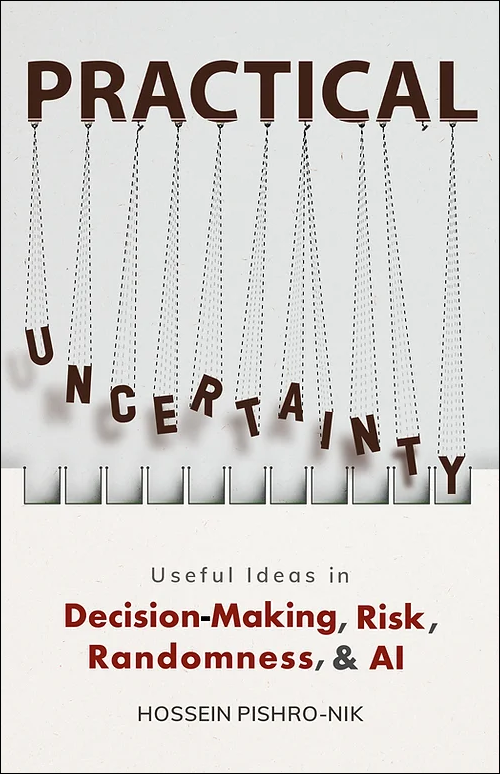## 1.4.4 Conditional Independence

As we mentioned earlier, almost any concept that is defined for probability can also be extended to conditional probability. Remember that two events $A$ and $B$ are independent if $$P(A \cap B)=P(A)P(B), \hspace{10pt} \textrm{or equivalently, } P(A|B)=P(A).$$ We can extend this concept to conditionally independent events. In particular,

Definition

Two events $A$ and $B$ are conditionally independent given an event $C$ with $P(C)>0$ if $$\hspace{100pt}P(A \cap B|C)=P(A|C)P(B|C) \hspace{100pt} (1.8)$$

Recall that from the definition of conditional probability, $$P(A|B)=\frac{P(A \cap B)}{P(B)},$$ if $P(B)>0$. By conditioning on $C$, we obtain $$P(A|B,C)=\frac{P(A \cap B|C)}{P(B|C)}$$ if $P(B|C), P(C) \neq 0$. If $A$ and $B$ are conditionally independent given $C$, we obtain

 $P(A | B,C)$ $=\frac{P(A \cap B|C)}{P(B|C)}$ $=\frac{P(A|C)P(B|C)}{P(B|C)}$ $=P(A|C)$.

Thus, if $A$ and $B$ are conditionally independent given $C$, then $$\hspace{100pt}P(A | B,C)=P(A|C) \hspace{100pt} (1.9)$$ Thus, Equations 1.8 and 1.9 are equivalent statements of the definition of conditional independence. Now let's look at an example.

Example

A box contains two coins: a regular coin and one fake two-headed coin ($P(H)=1$). I choose a coin at random and toss it twice. Define the following events.

• A= First coin toss results in an $H$.
• B= Second coin toss results in an $H$.
• C= Coin 1 (regular) has been selected.
Find $P(A|C), P(B|C), P(A \cap B|C), P(A), P(B),$ and $P(A \cap B)$. Note that $A$ and $B$ are NOT independent, but they are conditionally independent given $C$.

• Solution
• We have $P(A|C)=P(B|C)=\frac{1}{2}$. Also, given that Coin 1 is selected, we have $P(A \cap B|C)=\frac{1}{2}.\frac{1}{2}=\frac{1}{4}$. To find $P(A), P(B),$ and $P(A \cap B)$, we use the law of total probability:

 $P(A)$ $=P(A|C)P(C)+P(A|C^c)P(C^c)$ $=\frac{1}{2}\cdot \frac{1}{2} + 1\cdot \frac{1}{2}$ $=\frac{3}{4}$.

Similarly, $P(B)=\frac{3}{4}$. For $P(A \cap B)$, we have

 $P(A \cap B)$ $= P(A \cap B|C)P(C)+P(A \cap B|C^c)P(C^c)$ $=P(A|C)P(B|C)P(C)+P(A|C^c)P(B|C^c)P(C^c)$ $\hspace{120pt} \textrm{ (by conditional independence of$A$and$B$)}$ $=\frac{1}{2}\cdot \frac{1}{2}\cdot \frac{1}{2} + 1\cdot 1\cdot \frac{1}{2}$ $=\frac{5}{8}$.

As we see, $P(A \cap B)=\frac{5}{8}\neq P(A)P(B)=\frac{9}{16}$, which means that $A$ and $B$ are not independent. We can also justify this intuitively. For example, if we know $A$ has occurred (i.e., the first coin toss has resulted in heads), we would guess that it is more likely that we have chosen Coin 2 than Coin 1. This in turn increases the conditional probability that $B$ occurs. This suggests that $A$ and $B$ are not independent. On the other hand, given $C$ (Coin 1 is selected), $A$ and $B$ are independent.

One important lesson here is that, generally speaking, conditional independence neither implies (nor is it implied by) independence. Thus, we can have two events that are conditionally independent but they are not unconditionally independent (such as $A$ and $B$ above). Also, we can have two events that are independent but not conditionally independent, given an event $C$. Here is a simple example regarding this case. Consider rolling a die and let $$A=\{1,2\},$$ $$B=\{2,4,6\},$$ $$C=\{1,4\}.$$ Then, we have $$P(A)=\frac{1}{3}, P(B)=\frac{1}{2};$$ $$P(A \cap B)=\frac{1}{6}=P(A)P(B).$$ Thus, $A$ and $B$ are independent. But we have $$P(A|C)=\frac{1}{2}, P(B|C)=\frac{1}{2};$$ $$P(A \cap B|C)=P(\{2\}|C)=0.$$ Thus $$P(A \cap B|C) \neq P(A|C)P(B|C),$$ which means $A$ and $B$ are not conditionally independent given $C$.

 The print version of the book is available on Amazon.Practical uncertainty: Userful Ideas in Decision-Making, Risk, Randomness, & AI